# High School Chemistry : Identifying Acids and Bases

## Example Questions

### Example Question #1 : Identifying Acids And Bases

Which of the following will result when a base and an acid react with one another?

Possible Answers: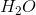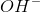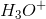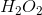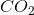Correct answer:Explanation:

Acids are able to donate protons whereas bases can donate hydroxyl groups. The general acid-base reaction will be a double-replacement reaction in which the proton of the acid binds the hydroxide of thee base, and the cation of the base binds the anion of the acid to form a salt. The products of this reaction are a salt and water. This is called a neutralization reaction.

### Example Question #1 : Identifying Acids And Bases

What is the conjugate base of nitric acid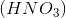?

Possible Answers: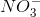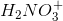Correct answer:Explanation:

Every acid has a conjugate base and every base has a conjugate acid. For any acid, the conjugate base is the negatively charged ion that is created when the acid dissociates in solution.

Nitric acid dissociates in solution based on the following reaction: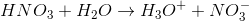The nitrate ion,, is created following the dissociation of nitric acid. This means that the nitrate ion is the conjugate base of nitric acid.

### Example Question #3 : Identifying Acids And Bases

Which of the following salts will result in an acidic solution?

Possible Answers: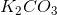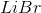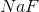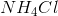Correct answer:Explanation:

A salt will dissolve in water completely, existing as the individual ions that make up the compound. These ions can be thought of as the conjugate bases and acids that result from the dissociation of the reactant acids and bases. If the conjugate acid of a weak base is present in the solution, then it will become deprotonated, releasing protons into the water and lowering the pH. To answer this question, we are looking for a salt in which one of the ions created is a conjugate acid of a weak base. Let's look at a few examples in order to find the right answer:

1.will dissociate into sodium and fluoride ions. Sodium is the metal found on sodium hydroxide. Since sodium hydroxide is considered a strong base, the sodium ions will NOT attach to any hydroxide ions in solution. Fluoride ions, on the other hand, are the conjugate base of hydrofluoric acid, a weak acid. This means that some of the fluoride ions will attach to protons in solution, effectively raising the pH. This results in a basic solution.

2.is an example of a salt that will result in a neutral solution. Because lithium ions come from the strong base lithium hydroxide, and bromide ions come from the strong acid hydrobromic acid, neither of these ions will be involved in an acid/base reaction. This results in a solution with a pH of 7.has two ions: ammonium ions and chloride ions. Chloride ions are the conjugate base of hydrochloric acid, a very strong acid. Ammonium ions, however, are the conjugate acid of ammonia, a weak base. This means that some of the ammonium ions will become deprotonated, and release protons into the solution. this results in an acidic solution.

### Example Question #4 : Identifying Acids And Bases

Which of the following aqueous compounds is a Brønsted-Lowry acid?

Possible Answers: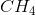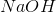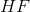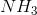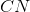Correct answer:Explanation:

A Brønsted-Lowry acid is an ionic compound that donates a proton, when the compound is placed in water.

HF and NaOH are the only ionic compounds of the given answer options; all the others are covalent compounds. When dissolved in water, only HF will donate protons.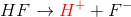Thus, HF is the only given Brønsted-Lowry acid.

### Example Question #5 : Identifying Acids And Bases

Which of the following is not a strong acid?

Possible Answers: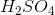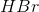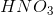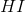Correct answer:Explanation:

An acid is classified as strong if it completely dissociates into ions in water. Some examples of common strong acids: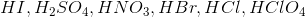is not a strong acid because it doesn't ionize completely in solution.

### Example Question #6 : Identifying Acids And Bases

Which of the following compounds may be classified as an Arrhenius acid?

Possible Answers: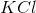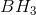Correct answer:Explanation:

By definition, and Arrhenius acid will dissociate in water to release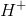.will dissociate intoand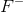in solution. The increased concentration ofcauses a drop in pH of the solution. In general, if a hydrogen atom is bound to a very electronegative atom, like in the case of, fluorine tends to take the electron away from hydrogen, resulting in ions.

### Example Question #7 : Identifying Acids And Bases

Which of the following is not an acid?

Possible Answers: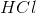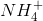Correct answer:Explanation:or ammonia is a complicated molecule. It's a kind of molecule that we call amphoteric, meaning that it can be an acid or a base. However, because ammonia plays the role of a very weak base, it's mostly thought of as having basic qualities. It has the ability to bind with acids to create an ammonium salt or act as a proton acceptor and become ammonium (acid).

Also, commonly, acids have the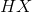format, whererepresents a halogen. In another construct, acids ideally have protons that they can donate - this can be observed in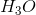and.

### Example Question #8 : Identifying Acids And Bases

Which of the following is not a base?

Possible Answers: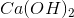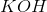Correct answer:Explanation:

Commonly, bases have the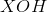format, whereis any metallic element from the first two columns of the periodic table. This is seen with, and. The remaining two options are essentially different "versions" of the same molecule - one just happens to be the protonated form ().

Whileis technically amphoteric, it's more so thought of in terms of its basic qualities. When it does act as an acid, it's a very weak acid. Ammonia can easily become ammonium, a proton donator, due to the lone pair of electrons that continue to orbit around the nitrogen center.

### All High School Chemistry Resources